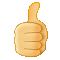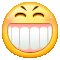# 【10】凤凰眼泪与格兰芬多之剑

## 用户评论(92)展示条数：20条20条50条100条

x x x x x x x x x x x x x x x x x x x x x x x x x x x x x x x x x x x x x x x x x x x x x x x x x x x x x x x x x x x x x x x x x x x👍🏻👍🏻👍🏻👍🏻👍🏻👍🏻👍🏻👍🏻👍🏻👍🏻👍🏻👍🏻👍🏻👍🏻👍🏻👍🏻👍🏻👍🏻👍🏻👍🏻👍🏻👍🏻👍🏻👍🏻👍🏻👍🏻👍🏻👍🏻👍🏻👍🏻👍🏻

∧＿∧ （・∀・） ⊂　⊂　） ㄑ　ㄑ　ㄑ （＿（＿） ━─ ,. ━─ ┏┯┯┯┯┯┓3 ┗┷┷┷┷┷┛ ((`'-"` `""-'`)) 　　 )　-　　-　 ( 　　/　 (o _ o) 　\ 　　\　　( 0 )　　/ 　_'-.._　'='　_..-'_ /`;#'#'#.　-.　#'#'#;`\ \_))　　　'#'　　　((_/ #.　　☆　☆　☆　　.# '#.　　我爱你!　　.#' 　/'#.　　　　　.#'\ 　_\\'#.　　　.#'//_ 　　(((___)'#'(___))) 　∧＿∧ （・∀・） （　∪　っ 　)　)　) （＿（＿） ！

∧＿∧ （・∀・） ⊂　⊂　） ㄑ　ㄑ　ㄑ （＿（＿） ━─ ,. ━─ ┏┯┯┯┯┯┓3 ┗┷┷┷┷┷┛ ((`'-"` `""-'`)) 　　 )　-　　-　 ( 　　/　 (o _ o) 　\ 　　\　　( 0 )　　/ 　_'-.._　'='　_..-'_ /`;#'#'#.　-.　#'#'#;`\ \_))　　　'#'　　　((_/ #.　　☆　☆　☆　　.# '#.　　我爱你!　　.#' 　/'#.　　　　　.#'\ 　_\\'#.　　　.#'//_ 　　(((___)'#'(___))) 　∧＿∧ （・∀・） （　∪　っ 　)　)　) （＿（＿） ！

H真神奇